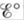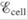# What are concentration cells? What is in a concentration cell? What is the driving force for a concentration cell to produce a voltage? Is the higher or the lower ion concentration solution present at the anode? When the anode ion concentration is decreased and/or the cathode ion concentration is increased, both give rise to larger cell potentials. Why? Concentration cells are commonly used to calculate the value of equilibrium constants for various reactions. For example, the silver concentration cell illustrated in Fig. 17-9 can be used to determine the K sp value for AgCl(s). To do so, NaCl is added to the anode compartment until no more precipitate forms. The [Cl − ] in solution is then determined somehow. What happens to when NaCl is added to the anode compartment? To calculate the K sp value, [Ag + ] must be calculated. Given the value of , how is [Ag + ] determined at the anode?### Chemistry: An Atoms First Approach

2nd Edition
Steven S. Zumdahl + 1 other
Publisher: Cengage Learning
ISBN: 9781305079243

#### Solutions

Chapter
Section### Chemistry: An Atoms First Approach

2nd Edition
Steven S. Zumdahl + 1 other
Publisher: Cengage Learning
ISBN: 9781305079243
Chapter 17, Problem 6RQ
Textbook Problem
1 views

## What are concentration cells? What isin a concentration cell? What is the driving force for a concentration cell to produce a voltage? Is the higher or the lower ion concentration solution present at the anode? When the anode ion concentration is decreased and/or the cathode ion concentration is increased, both give rise to larger cell potentials. Why? Concentration cells are commonly used to calculate the value of equilibrium constants for various reactions. For example, the silver concentration cell illustrated in Fig. 17-9 can be used to determine the Ksp value for AgCl(s). To do so, NaCl is added to the anode compartment until no more precipitate forms. The [Cl−] in solution is then determined somehow. What happens towhen NaCl is added to the anode compartment? To calculate the Ksp value, [Ag+] must be calculated. Given the value of, how is [Ag+] determined at the anode?

Interpretation Introduction

Interpretation:

The condition for common ion effect in concentration cell is given. The concept of concentration cell along with effect of common ion effect in the concentration cell is to be stated.

Concept introduction:

The difference in ion concentration in different compartments leads to the formation of the concentration cell.

The common ion effect also affects the value of the cell potential.

To determine: The definition of concentration cell; the value of E°cell in the concentration cell; driving force for a concentration cell to produce a voltage; concentration of ion at anode; effect of decreased concentration of ion at anode and/or increased concentration of ion at cathode over cell potentials; effect of addition of NaCl to the anode compartment (refer Fig.17-9); the value of [Ag+] at anode using the given Ecell .

### Explanation of Solution

A concentration cell is that type of cell which consists of same type of electrodes, but they differ in concentration.

When the transport of substance occurs from one half cell to another due to difference in concentration gradient there occurs generation of current. This leads to the formation of concentration cells.

They are of two types,

• Electrode concentration cell
• Electrolyte concentration cell

The value of Ecell is zero for the given concentration cell.

In a concentration cell, both the compartments are composed of the same type of component that makes Ecell zero.

The difference in ion concentration in both the compartments is the main driving force for the production of voltage in the concentration cell.

The voltage will generate in a concentration cell when there will be a difference of concentration of ion in the cathode and anode compartment. When the concentration of ions becomes equal in both the compartments, the value of cell potential comes out to be zero.

Lower ion concentration is present at the anode.

The expression for concentration cell comes out to be,

Ecell = 2.303RTnF log (acathodeaanode)

Where,

• R is the universal gas constant.
• T is temperature.
• n is the number of electrons transferred.
• F is the Faraday’s constant.
• acathode is the activity of the ions at the cathode.
• aanode is the activity of the ions at the anode.

This term will be positive only when the concentration of ion at anode is lower than that of ion concentration at cathode.

The value of Ecell is calculated by the formula,

Ecell = 2.303RTnF log (acathodeaanode)

When the concentration of ion is lower at anode and/or higher at cathode, the value of Ecell becomes positive

### Still sussing out bartleby?

Check out a sample textbook solution.

See a sample solution

#### The Solution to Your Study Problems

Bartleby provides explanations to thousands of textbook problems written by our experts, many with advanced degrees!

Get Started

Find more solutions based on key concepts
Type 2 diabetes often improves with a diet that is a. low in carbohydrates (less than 130 g per day). b. as low...

Nutrition: Concepts and Controversies - Standalone book (MindTap Course List)

25-43 What is the difference between miRNA AND siRNA?

Introduction to General, Organic and Biochemistry

What is genomics?

Human Heredity: Principles and Issues (MindTap Course List)

Why can’t a white dwarf have a mass greater than 1.4 solar masses?

Horizons: Exploring the Universe (MindTap Course List)

Which molecule does not form during glycolysis? a. NADH b. pyruvate c. FADH2 d. ATP

Biology: The Unity and Diversity of Life (MindTap Course List)

What is the escape speed of the Earth and of the Moon?

Physics for Scientists and Engineers: Foundations and Connections

What happens at each?

Oceanography: An Invitation To Marine Science, Loose-leaf Versin

A resistor R, inductor L, and capacitor C are connected in series to an AC source of rms voltage V and variable...

Physics for Scientists and Engineers, Technology Update (No access codes included)